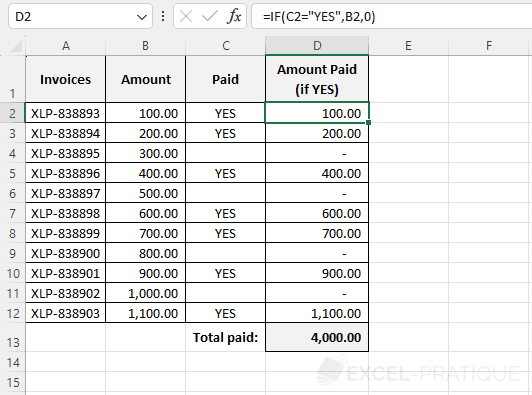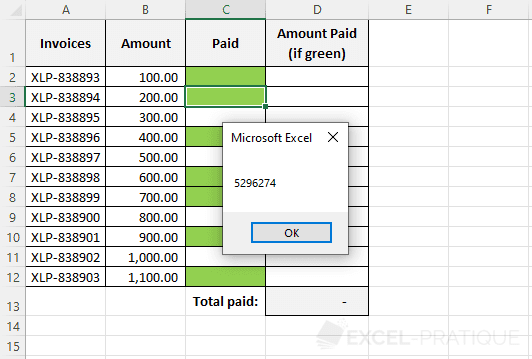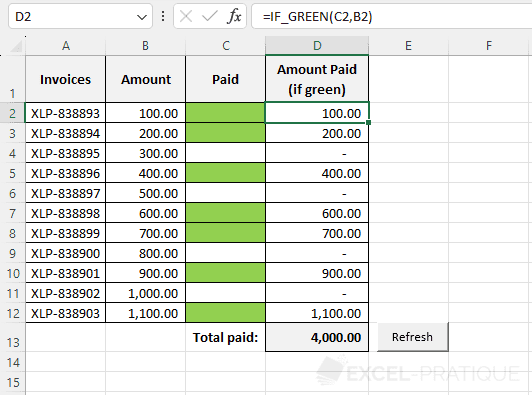VBA Course: Create a Custom Function

In this example, we will create a custom IF function that can be used on a calculation worksheet like any other function.

To display a value (B2) if a cell (C2) has the value ("YES"), we could use the IF function with this formula =IF(C2="YES",B2,0):Our goal in this case is to create a function that can do this =IF(C2 has a green background,B2,0) which we would write as follows: =IF_GREEN(C2,B2):Develop the custom function

Start by creating the function:

Function IF_GREEN(paid As Range, amount)

End Function

The arguments:

• paid As Range: the cell to test
• amount: the value to insert if TRUE

In this case, if the test is FALSE, the value will be 0 every time, so there's no reason to have an argument for that.

To test to see whether the color is correct, you can use a cell that contains the color that you want as a point of reference:

Function IF_GREEN(paid As Range, amount)

green_color = Sheets("Sheet1").Range("K1").Interior.color

End Function

But to avoid having to use another cell for this purpose, we'll use the number of the color that we're interested in here:

Function IF_GREEN(paid As Range, amount)

green_color = 5296274 'Green

End Function

To figure out a cell's background color number, select the cell and run this macro:

Sub test_color()
MsgBox ActiveCell.Interior.color
End SubNow all we have to do is test the color number using If:

Function IF_GREEN(paid As Range, amount)

Application.Volatile

green_color = 5296274 'Green

If paid.Interior.color = green_color Then 'If TRUE
IF_GREEN = amount
Else 'If FALSE
IF_GREEN = 0
End If

End Function

Our function is now ready to be used.

Application.Volatile tells us that the application is volatile (which is the case for the IF function). This means it should be recalculated each time a value is changed. For example, if you modify any of the amounts (or any other cell, in fact), the function will be recalculated and the correct amount will be displayed.

However, changing the background color does not trigger this recalculation. To force recalculation of the values, you can select an empty cell and press "Delete" or add an "Refresh" button that will cause everything to be updated when it is clicked:

Sub refresh_macro()
Application.Calculate
End SubExtras

Here is another way to write the same function:

Function IF_GREEN(paid As Range, amount)
Application.Volatile
IF_GREEN = 0 'If FALSE
If paid.Interior.color = 5296274 Then IF_GREEN = amount 'If TRUE
End Function

You can download the Excel file that contains this example here: if_green_function.zip